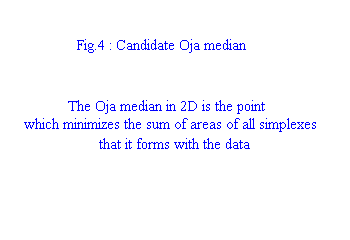Oja's Simplex Median (Oja 1983)

Consider p+1 points in Rp. These points form a simplex, which has a p-dimensional volume. For example, in R3 four points form a tetrahedron, and in R2 three points form a triangle whose area is "2-dimensional volume". Now consider a data set in Rp for which we seek the median. Oja proposed the following measure for a point X in Rp:

• for every subset of p points from the data set, form a simplex with X.
• sum together the volumes of each such simplex.
• the Oja simplex median is any point X* in Rp for which this sum is minimum.

Fig.4 shows all the simplexes that must be summed together for a given data set of five points and "candidate median point".Since one-dimensional volume is length, the Oja median reduces to the standard univariate median. In 1D, the Oja median minimizes the sum of distances to all data points, as does the L1. It is interesting that the Oja median need not be unique. An important feature is that it is affine invariant (invariant to rigid transformations, scaling and shearing). However, it has been found to have a "0%" breakdown point. Notice that if the data does not "span" the dimension of the space that it is in (ex: data on a line in R2 or data on a plane in R3), then it is possible to find simplexes with zero volume, even at infinity. This seems like an unrealistic case for pattern recognition though. At present I am not familiar with any algorithms that calculate the Oja median.

UPDATE

next : the halfspace median